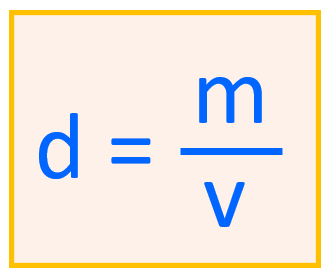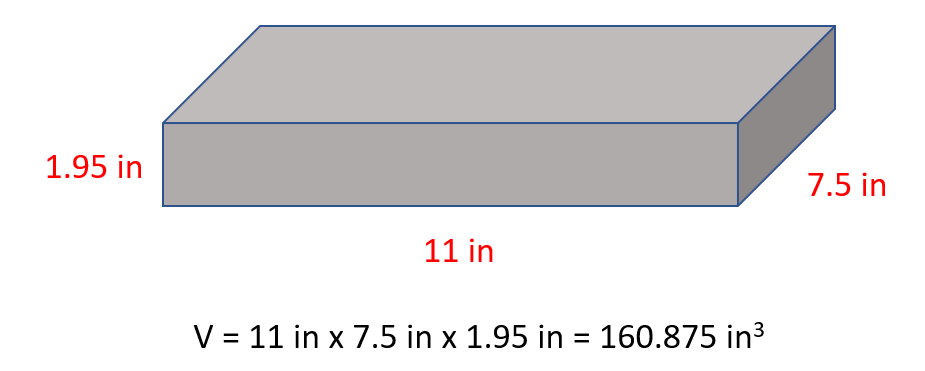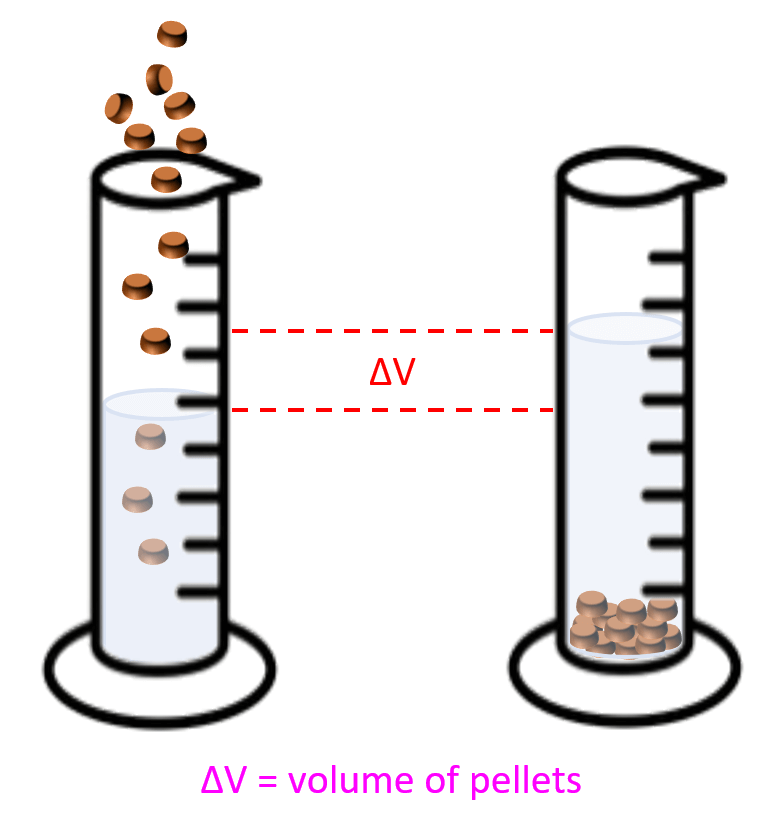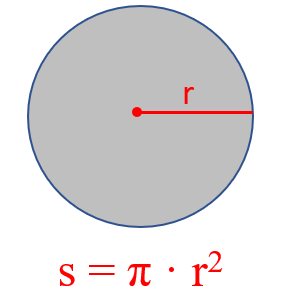## General Chemistry

In these practice problems, we will work on determining the density, volume, and the mass of different objects. First, density is calculated by the ratio of the mass and the volume of the object:For example, what is the density of a metal if its 2.35 g sample has a volume of 0.654 g/mL?

${\rm{d}}\;{\rm{ = }}\,\,\frac{{\rm{m}}}{{\rm{v}}}\,{\rm{ = }}\frac{{{\rm{2}}{\rm{.35}}\,{\rm{g}}}}{{{\rm{0}}{\rm{.654}}\,{\rm{mL}}}}\,{\rm{ = }}\;{\rm{3}}{\rm{.59}}\,{\rm{g/mL}}$

Sometimes the volume may not be given and there are two main scenarios here depending on if the object has a regular or irregular shape. So, let’s discuss them one by one.

# The density of Objects with Regular Shapes

The first example here would be the density of an object with a cubic shape. For example, a wood block with a length of 11 in, 7.5 in width and 1.95 in thickness weighs 4.93 lb. Calculate density of the block in lb/in3.

The mass is given and therefore, the only thing missing is the volume of the block which we find by multiplying all the sides:And now, we can determine the density:

${\rm{d}}\;{\rm{ = }}\,\,\frac{{\rm{m}}}{{\rm{v}}}\,{\rm{ = }}\frac{{{\rm{4}}{\rm{.93}}\,{\rm{lb}}}}{{{\rm{160}}{\rm{.875}}\,{\rm{i}}{{\rm{n}}^{\rm{3}}}}}\,{\rm{ = }}\;{\rm{0}}{\rm{.031}}\,{\rm{lb/i}}{{\rm{n}}^{\rm{3}}}$

# The density of Objects with Irregular Shapes

The most common example here is the one where the density of metal pellets needs to be determined. For example, a sample containing 15.4 g of metal pellets is poured into a graduated cylinder initially containing 12.0 mL of water, causing the water level in the cylinder to rise to 16.2 mL. Calculate the density of the metal.

What you need to visualize in these problems, is that the volume of pellets or anything else that was added to water, is equal to the volume of the water displaced:So, in this case, the volume of the pellets would be:

16.2 – 12.0 = 4.20 mL

Therefore, the density is the ratio of the mass and this difference in initial and final volumes:

${\rm{d}}\;{\rm{ = }}\,\,\frac{{\rm{m}}}{{\rm{v}}}\,{\rm{ = }}\frac{{{\rm{15}}{\rm{.4}}\,{\rm{g}}}}{{{\rm{4}}{\rm{.20}}\,{\rm{mL}}}}\,{\rm{ = }}\;{\rm{3}}{\rm{.67}}\,{\rm{g/mL}}$

# Density When the Units are Different

Another type of problem is when the initial units are different than what they are asked to be in the answer. For example, determine the density of a plastic in g/cm3 if a 1.39-lb piece occupies 6.48 in3 volume.

First, we can calculate the density in lb/in3 and then convert the units to g/cm3.

${\rm{d}}\;{\rm{ = }}\,\,\frac{{\rm{m}}}{{\rm{v}}}\,{\rm{ = }}\frac{{{\rm{1}}{\rm{.39}}\,{\rm{lb}}}}{{{\rm{6}}{\rm{.48}}\,{\rm{i}}{{\rm{n}}^{\rm{3}}}}}\,{\rm{ = }}\;{\rm{0}}{\rm{.2145}}\,{\rm{lb/i}}{{\rm{n}}^{\rm{3}}}$

Now, remember, for converting two units, we treat them like separate units and do the conversions one by one. Check the “Multi-Step Unit Conversion” section here for more details.

${\rm{0}}{\rm{.2145}}\;\frac{{\cancel{{{\rm{lb}}}}}}{{\cancel{{{\rm{i}}{{\rm{n}}^{\rm{3}}}}}}}\,{\rm{ \times }}\,\frac{{{\rm{453}}{\rm{.6}}\,{\rm{g}}}}{{{\rm{1}}\;\cancel{{{\rm{lb}}}}}}\,{\rm{ \times }}\,\frac{{{\rm{(1}}\,\cancel{{{\rm{in}}{{\rm{)}}^{\rm{3}}}}}}}{{{{{\rm{(2}}{\rm{.54}}\,{\rm{cm)}}}^{\rm{3}}}}}\,{\rm{ = }}\;{\rm{5}}{\rm{.94}}\,{\rm{g/c}}{{\rm{m}}^{\rm{3}}}\,\,$

So, in the first part, we converted pounds to grams, and the second multiplication was to convert in3 to cm3. Remember, you need to apply the exponent to both the number and the unit when converting units raised to a power (Converting Units Raised to Power).

# The density of a Cylinder

Another common question is determining the density of a cylinder. What you need to remember here is the formula that may not be given:If you forget it, try to remember the formula for the surface of circle:Now you can visualize the cylinder as a stack of multiple circles and therefore, its volume is the product of the circle’s surface and the height of the cylinder.For example, a plastic cylinder has a length of 8.52 in, a radius of 2.34 in, and a mass of 5.60 lb. What is the density of the plastic in lb/in3?

The volume of a cylinder is calculated by the formula v = π · r· l, and therefore,

v = π · r· l = 3.14 x (2.34)in x 8.52 in = 146.488 in3

The density is the ratio of the mass and the calculated volume:

${\rm{d}}\;{\rm{ = }}\,\,\frac{{\rm{m}}}{{\rm{v}}}\,{\rm{ = }}\frac{{{\rm{5}}{\rm{.60}}\,{\rm{lb}}}}{{{\rm{146}}{\rm{.488}}\,{\rm{i}}{{\rm{n}}^{\rm{3}}}}}\,{\rm{ = }}\;{\rm{0}}{\rm{.0382}}\,{\rm{lb/i}}{{\rm{n}}^{\rm{3}}}$

# Mass and Volume from Density

The formula for the density can be rearranged to get an expression for the mass or the volume. For example, what is the mass of a metal block with a density of 9.25 g/ml if it occupies 14.6 cm3 volume?

Rearranging the formula for density, we ger that the mass if the product of the volume and density:

m = d x v

Therefore,

${\rm{m}}\;{\rm{ = }}\,{\rm{d}}\,{\rm{ \times }}\,{\rm{v}}\,{\rm{ = }}\,{\rm{9}}{\rm{.25}}\,\frac{{\rm{g}}}{{\cancel{{{\rm{mL}}}}}}\;{\rm{ \times }}\,{\rm{14}}{\rm{.6}}\,\cancel{{{\rm{mL}}}}\,{\rm{ = }}\;{\rm{135}}\;{\rm{g}}$

Notice that 1 mL = 1cm3 that is why we replaced cm3 by ml for the volume and canceled them with the density units.

The volume is the ratio of the mass and density. For example, what is the volume of 154 g bromine in milliliters if it has a density of 3.10 g/cm3?

${\rm{v}}\;{\rm{ = }}\,\frac{{\rm{m}}}{{\rm{d}}}\,{\rm{ = }}\,\frac{{{\rm{154}}\,{\rm{g}}}}{{{\rm{3}}{\rm{.10}}\,{\rm{c}}{{\rm{m}}^{\rm{3}}}}}\,{\rm{ = }}\;{\rm{49}}{\rm{.7}}\,{\rm{g/c}}{{\rm{m}}^{\rm{3}}}$

Check Also

#### Practice

1.

How many dozen eggs are in 15,652 eggs?

This content is available to registered users only. Click here to Register! By joining Chemistry Steps, you will gain instant access to the answers and solutions for all the Practice Problems and the powerful set of General Chemistry 1 and 2 Summary Study Guides.
Solution
This content is available to registered users only. Click here to Register! By joining Chemistry Steps, you will gain instant access to the answers and solutions for all the Practice Problems and the powerful set of General Chemistry 1 and 2 Summary Study Guides.
2.

How many years are in 5,489 days?

This content is available to registered users only. Click here to Register! By joining Chemistry Steps, you will gain instant access to the answers and solutions for all the Practice Problems and the powerful set of General Chemistry 1 and 2 Summary Study Guides.
Solution
This content is available to registered users only. Click here to Register! By joining Chemistry Steps, you will gain instant access to the answers and solutions for all the Practice Problems and the powerful set of General Chemistry 1 and 2 Summary Study Guides.
3.

How many weeks are in 2.5 centuries? (1 yr. = 52 weeks)

This content is available to registered users only. Click here to Register! By joining Chemistry Steps, you will gain instant access to the answers and solutions for all the Practice Problems and the powerful set of General Chemistry 1 and 2 Summary Study Guides.
Solution
This content is available to registered users only. Click here to Register! By joining Chemistry Steps, you will gain instant access to the answers and solutions for all the Practice Problems and the powerful set of General Chemistry 1 and 2 Summary Study Guides.
4.

Would 550 m2 of fabric be enough to upholster 200 chairs if each requires 35.4 ft2 fabric?

This content is available to registered users only. Click here to Register! By joining Chemistry Steps, you will gain instant access to the answers and solutions for all the Practice Problems and the powerful set of General Chemistry 1 and 2 Summary Study Guides.
Solution
This content is available to registered users only. Click here to Register! By joining Chemistry Steps, you will gain instant access to the answers and solutions for all the Practice Problems and the powerful set of General Chemistry 1 and 2 Summary Study Guides.
5.

A 20.0-mL sample of a liquid has a mass of 17.8 g. What is the liquid’s density in grams per milliliter?

This content is available to registered users only. Click here to Register! By joining Chemistry Steps, you will gain instant access to the answers and solutions for all the Practice Problems and the powerful set of General Chemistry 1 and 2 Summary Study Guides.
Solution
This content is available to registered users only. Click here to Register! By joining Chemistry Steps, you will gain instant access to the answers and solutions for all the Practice Problems and the powerful set of General Chemistry 1 and 2 Summary Study Guides.
6.

A sample containing 31.25 g of metal pellets is poured into a graduated cylinder initially containing 11.9 mL of water, causing the water level in the cylinder to rise to 18.7 mL. Calculate the density of the metal.

This content is available to registered users only. Click here to Register! By joining Chemistry Steps, you will gain instant access to the answers and solutions for all the Practice Problems and the powerful set of General Chemistry 1 and 2 Summary Study Guides.
Solution
This content is available to registered users only. Click here to Register! By joining Chemistry Steps, you will gain instant access to the answers and solutions for all the Practice Problems and the powerful set of General Chemistry 1 and 2 Summary Study Guides.
7.

What is the mass of a 2.85 L sample of a liquid that has a density of 0.954 g/mL?

This content is available to registered users only. Click here to Register! By joining Chemistry Steps, you will gain instant access to the answers and solutions for all the Practice Problems and the powerful set of General Chemistry 1 and 2 Summary Study Guides.
Solution
This content is available to registered users only. Click here to Register! By joining Chemistry Steps, you will gain instant access to the answers and solutions for all the Practice Problems and the powerful set of General Chemistry 1 and 2 Summary Study Guides.
8.

What is the volume of 100. g bromine in milliliters if it has a density of 3.10 g/cm3?

This content is available to registered users only. Click here to Register! By joining Chemistry Steps, you will gain instant access to the answers and solutions for all the Practice Problems and the powerful set of General Chemistry 1 and 2 Summary Study Guides.
Solution
This content is available to registered users only. Click here to Register! By joining Chemistry Steps, you will gain instant access to the answers and solutions for all the Practice Problems and the powerful set of General Chemistry 1 and 2 Summary Study Guides.
9.

Complete the missing data for the density, mass, and volume in the following table:

 Mass Volume Density 2.35 g 0.035 L X g/mL X lb 356 mL 1.56 g/cm3 14.6 kg X gal 4.81 g/mL

a)
This content is available to registered users only. Click here to Register! By joining Chemistry Steps, you will gain instant access to the answers and solutions for all the Practice Problems and the powerful set of General Chemistry 1 and 2 Summary Study Guides.
b)
This content is available to registered users only. Click here to Register! By joining Chemistry Steps, you will gain instant access to the answers and solutions for all the Practice Problems and the powerful set of General Chemistry 1 and 2 Summary Study Guides.
c)
This content is available to registered users only. Click here to Register! By joining Chemistry Steps, you will gain instant access to the answers and solutions for all the Practice Problems and the powerful set of General Chemistry 1 and 2 Summary Study Guides.
Solution
This content is available to registered users only. Click here to Register! By joining Chemistry Steps, you will gain instant access to the answers and solutions for all the Practice Problems and the powerful set of General Chemistry 1 and 2 Summary Study Guides.
10.

How many kilograms of honey with a density of 1.40 kg/L are there in a gallon container?

This content is available to registered users only. Click here to Register! By joining Chemistry Steps, you will gain instant access to the answers and solutions for all the Practice Problems and the powerful set of General Chemistry 1 and 2 Summary Study Guides.
Solution
This content is available to registered users only. Click here to Register! By joining Chemistry Steps, you will gain instant access to the answers and solutions for all the Practice Problems and the powerful set of General Chemistry 1 and 2 Summary Study Guides.
11.

The density of iron is 8.96 g/cm3. What is its density in pounds per cubic inch (lb/in3)?

This content is available to registered users only. Click here to Register! By joining Chemistry Steps, you will gain instant access to the answers and solutions for all the Practice Problems and the powerful set of General Chemistry 1 and 2 Summary Study Guides.
Solution
This content is available to registered users only. Click here to Register! By joining Chemistry Steps, you will gain instant access to the answers and solutions for all the Practice Problems and the powerful set of General Chemistry 1 and 2 Summary Study Guides.
12.

The density of iron is 7.86 g/cm3. What is the volume of 5.24 lb of iron expressed in cubic inches?

This content is available to registered users only. Click here to Register! By joining Chemistry Steps, you will gain instant access to the answers and solutions for all the Practice Problems and the powerful set of General Chemistry 1 and 2 Summary Study Guides.
Solution
This content is available to registered users only. Click here to Register! By joining Chemistry Steps, you will gain instant access to the answers and solutions for all the Practice Problems and the powerful set of General Chemistry 1 and 2 Summary Study Guides.
13.

A plastic cylinder has a length of 7.25 in, a radius of 1.26 in, and a mass of 841 g. What is the density of the plastic in g/cm3?